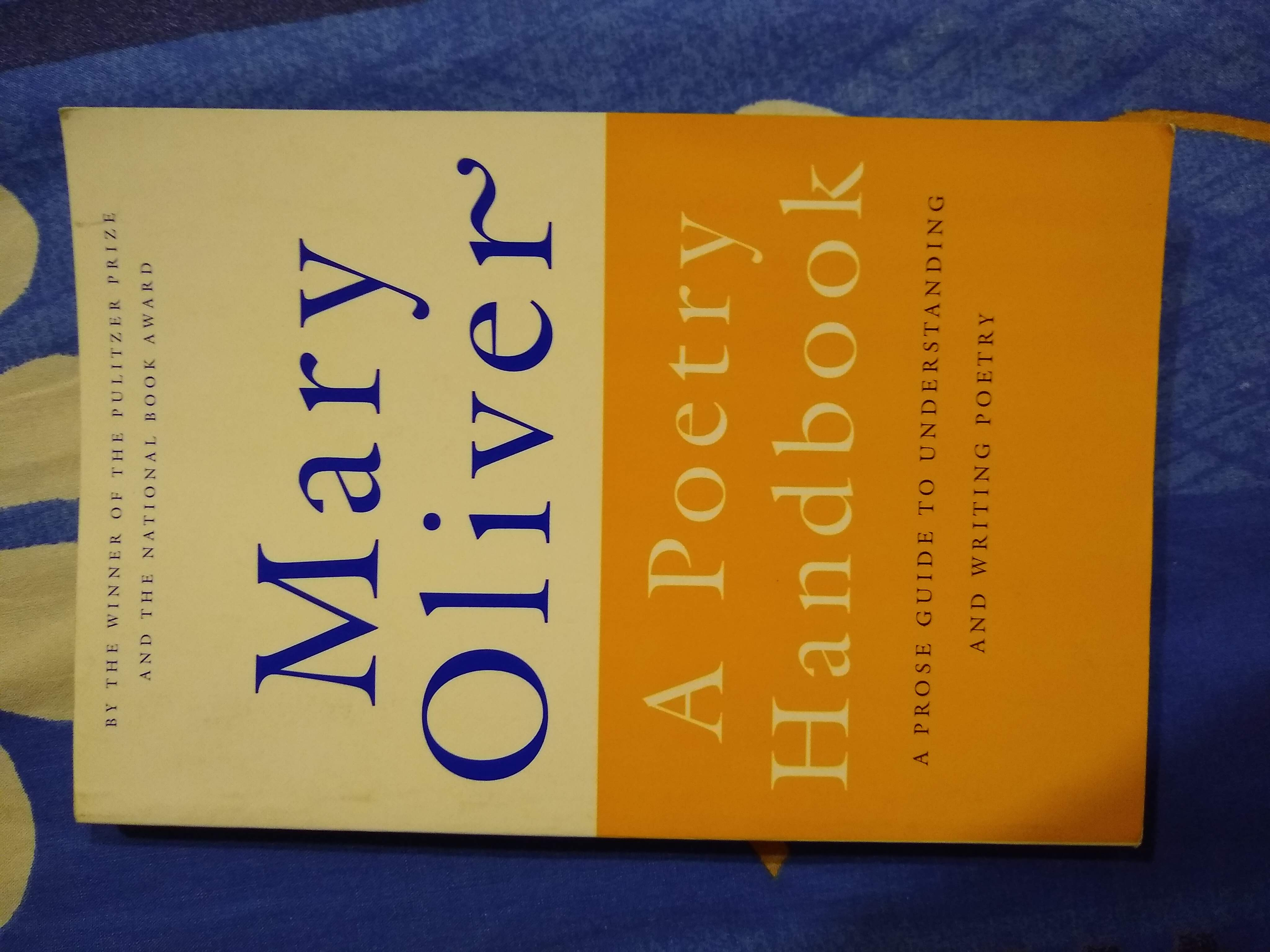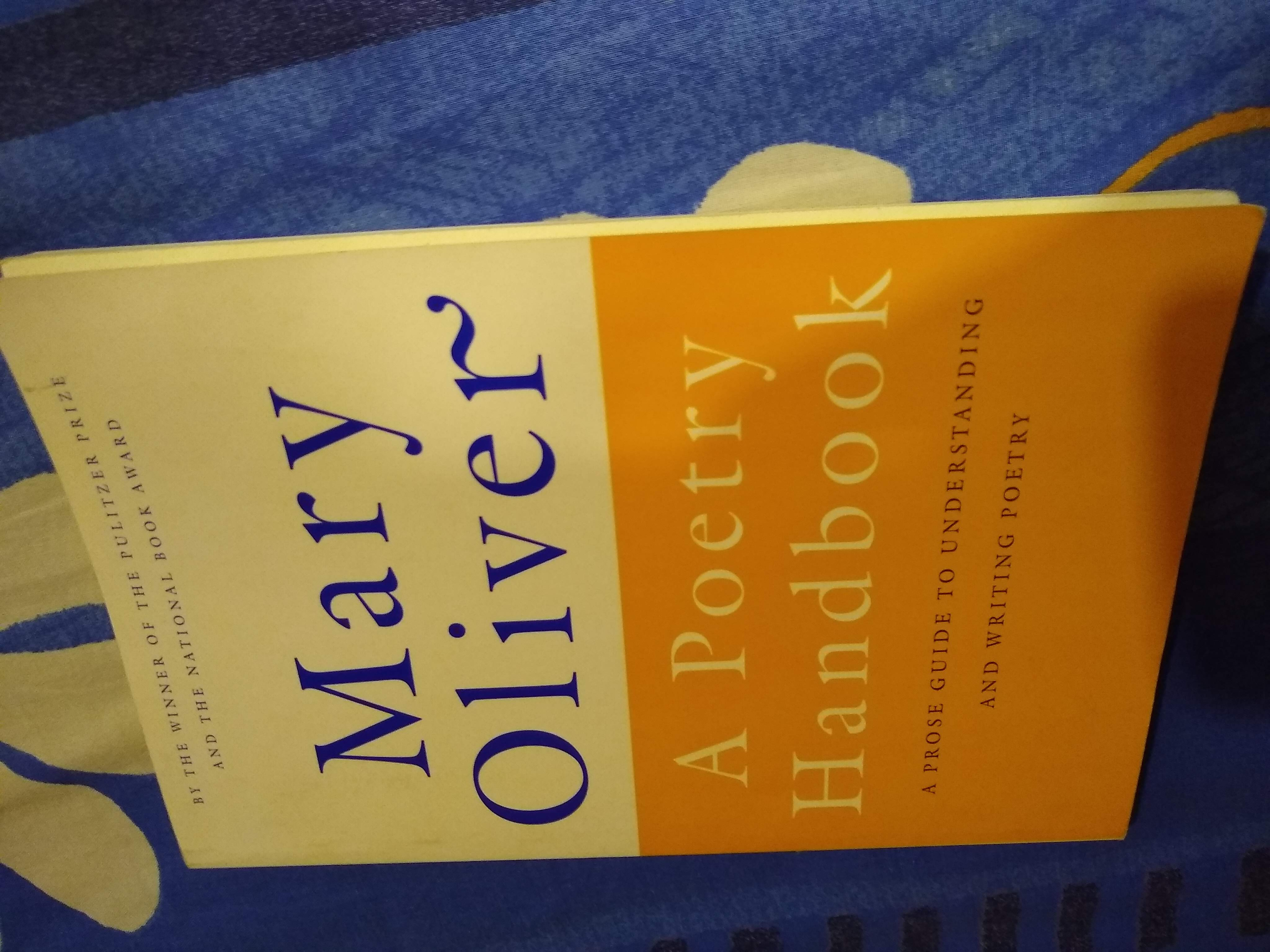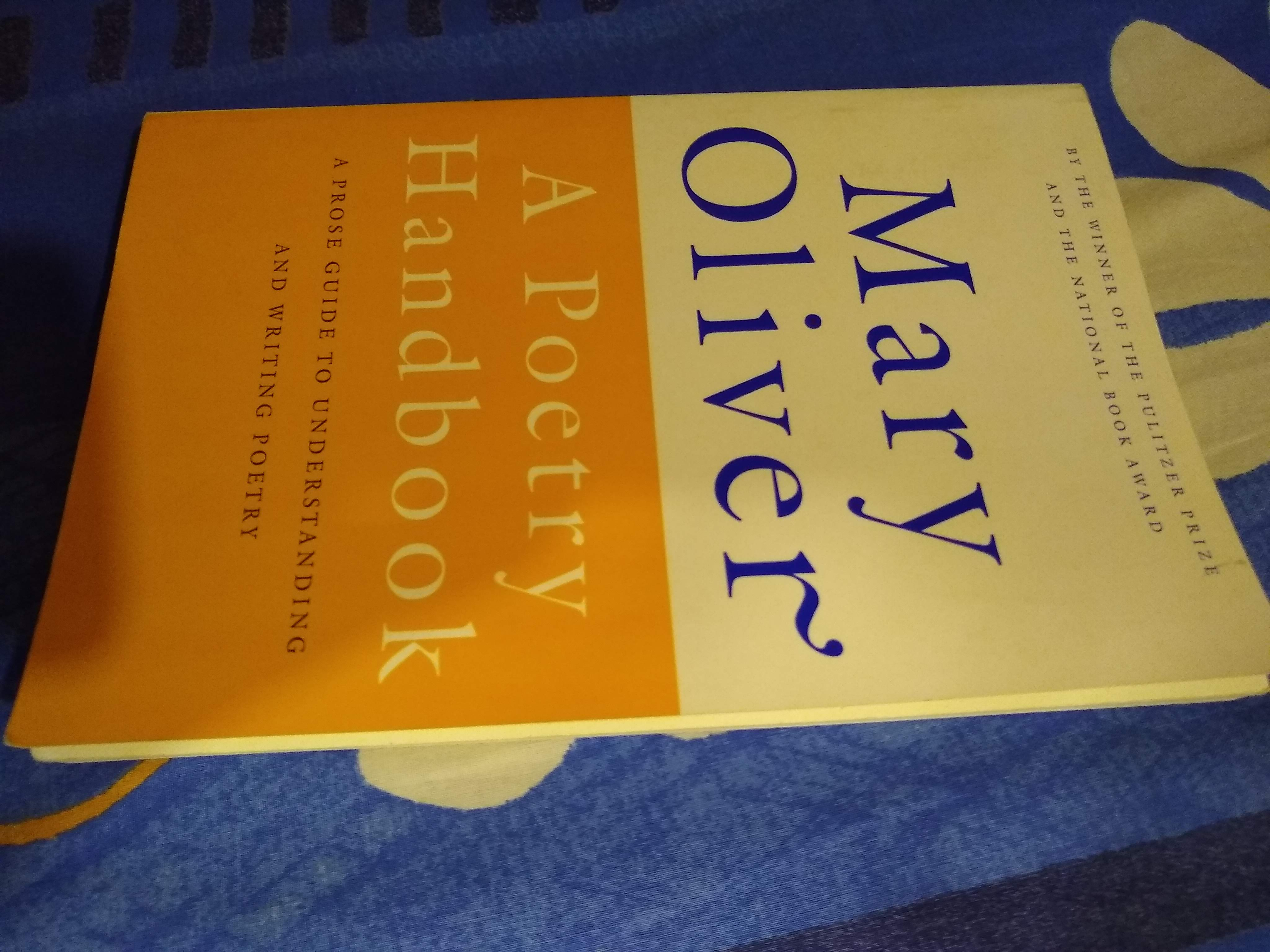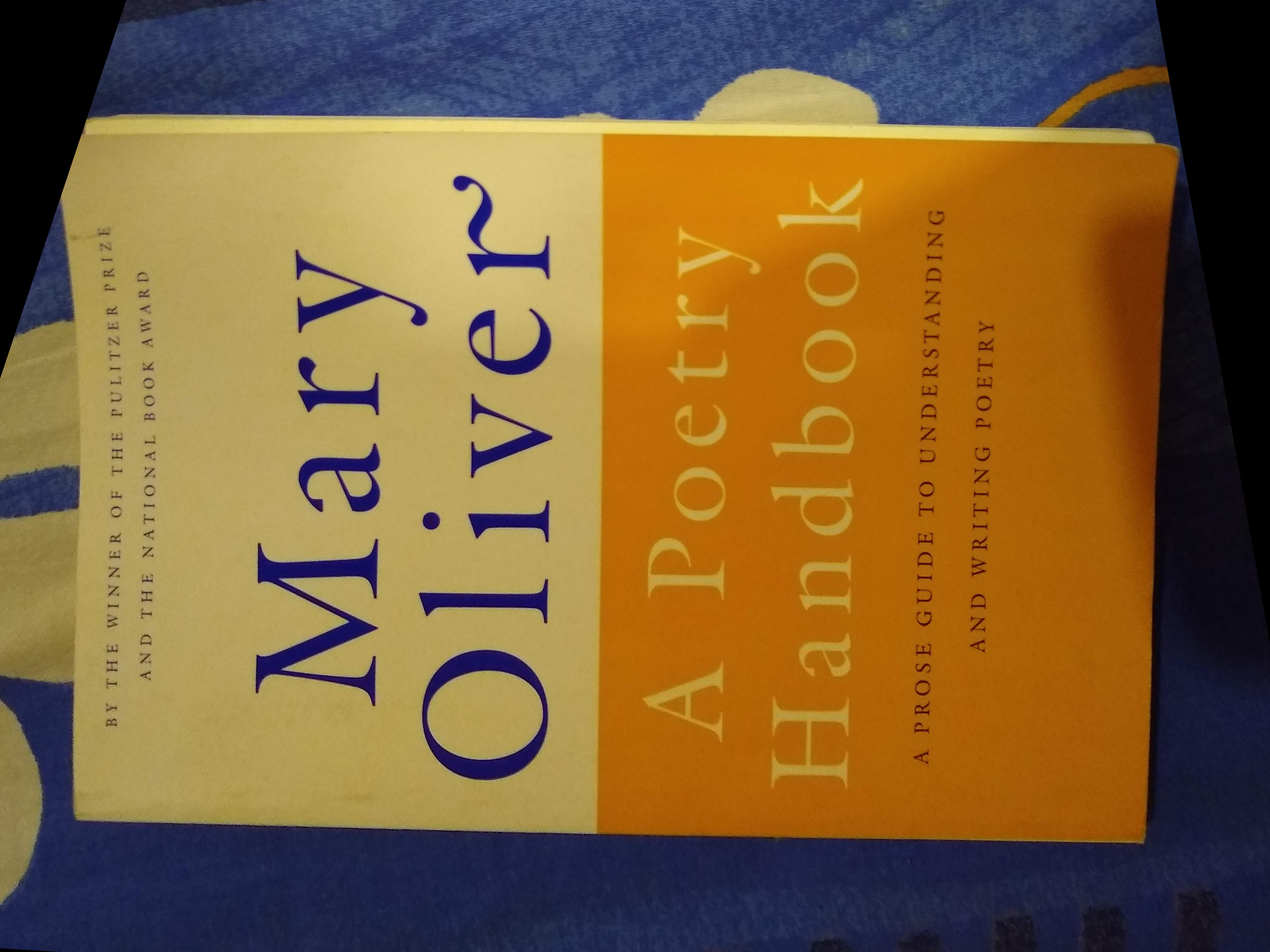# Image Registration using OpenCV | Python

Image registration is a digital image processing technique which helps us align different images of the same scene. For instance, one may click the picture of a book from various angles. Below are a few instances that show the diversity of camera angle.

Now, we may want to “align” a particular image to the same angle as a reference image. In the images above, one may consider the first image to be an “ideal” cover photo, while the second and third images do not serve well for book cover photo purposes. The image registration algorithm helps us align the second and third pictures to the same plane as the first one.How does image registration work?
Alignment can be looked at as a simple coordinate transform. The algorithm works as follows:

• Convert both images to grayscale.
• Match features from the image to be aligned, to the reference image and store the coordinates of the corresponding keypoints. Keypoints are simply the selected few points which are used to compute the transform (generally points that stand out), and descriptors are histograms of the image gradients to characterize the appearance of a keypoint. In this post, we use ORB (Oriented FAST and Rotated BRIEF) implementation in the OpenCV library, which provides us with both keypoints as well as their associated descriptors.
• Match the keypoints between the two images. In this post, we use BFMatcher, which is a brute force matcher.` BFMatcher.match()` retrieves the best match, while `BFMatcher.knnMatch()` retrieves top K matches, where K is specified by the user.
• Pick the top matches, and remove the noisy matches.
• Find the homomorphy transform.
• Apply this transform to the original unaligned image to get the output image.

Applications of Image Registration –
Some of the useful applications of image registration include:

• Stiching various scenes (which may or may not have the same camera alignment) together to form a continuous panaromic shot.
• Aligning camera images of documents to a standard alignment to create realistic scanned documents.
• Aligning medical images for better observation and analysis.

Below is the code for image registration. We have aligned the second image with reference to the third image.

 `import` `cv2 ` `import` `numpy as np ` ` `  `# Open the image files. ` `img1_color ``=` `cv2.imread(``"align.jpg"``)  ``# Image to be aligned. ` `img2_color ``=` `cv2.imread(``"ref.jpg"``)    ``# Reference image. ` ` `  `# Convert to grayscale. ` `img1 ``=` `cv2.cvtColor(img1_color, cv2.COLOR_BGR2GRAY) ` `img2 ``=` `cv2.cvtColor(img2_color, cv2.COLOR_BGR2GRAY) ` `height, width ``=` `img2.shape ` ` `  `# Create ORB detector with 5000 features. ` `orb_detector ``=` `cv2.ORB_create(``5000``) ` ` `  `# Find keypoints and descriptors. ` `# The first arg is the image, second arg is the mask ` `#  (which is not reqiured in this case). ` `kp1, d1 ``=` `orb_detector.detectAndCompute(img1, ``None``) ` `kp2, d2 ``=` `orb_detector.detectAndCompute(img2, ``None``) ` ` `  `# Match features between the two images. ` `# We create a Brute Force matcher with  ` `# Hamming distance as measurement mode. ` `matcher ``=` `cv2.BFMatcher(cv2.NORM_HAMMING, crossCheck ``=` `True``) ` ` `  `# Match the two sets of descriptors. ` `matches ``=` `matcher.match(d1, d2) ` ` `  `# Sort matches on the basis of their Hamming distance. ` `matches.sort(key ``=` `lambda` `x: x.distance) ` ` `  `# Take the top 90 % matches forward. ` `matches ``=` `matches[:``int``(``len``(matches)``*``90``)] ` `no_of_matches ``=` `len``(matches) ` ` `  `# Define empty matrices of shape no_of_matches * 2. ` `p1 ``=` `np.zeros((no_of_matches, ``2``)) ` `p2 ``=` `np.zeros((no_of_matches, ``2``)) ` ` `  `for` `i ``in` `range``(``len``(matches)): ` `  ``p1[i, :] ``=` `kp1[matches[i].queryIdx].pt ` `  ``p2[i, :] ``=` `kp2[matches[i].trainIdx].pt ` ` `  `# Find the homography matrix. ` `homography, mask ``=` `cv2.findHomography(p1, p2, cv2.RANSAC) ` ` `  `# Use this matrix to transform the ` `# colored image wrt the reference image. ` `transformed_img ``=` `cv2.warpPerspective(img1_color, ` `                    ``homography, (width, height)) ` ` `  `# Save the output. ` `cv2.imwrite(``'output.jpg'``, transformed_img) `

Output:My Personal Notes arrow_drop_upCheck out this Author's contributed articles.

If you like GeeksforGeeks and would like to contribute, you can also write an article using contribute.geeksforgeeks.org or mail your article to contribute@geeksforgeeks.org. See your article appearing on the GeeksforGeeks main page and help other Geeks.

Please Improve this article if you find anything incorrect by clicking on the "Improve Article" button below.

Article Tags :

1

Please write to us at contribute@geeksforgeeks.org to report any issue with the above content.